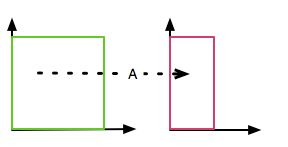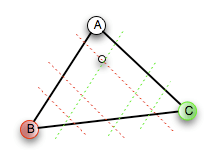# The Sierpinski Gasket by AffineSo, in my last post, I promised to explain how the chaos game is is an attractor for the Sierpinski triangle. It’s actually pretty simple. First, though, we’ll introduce the idea of an affine transformation. Affine transformations aren’t strictly necessary for understanding the Chaos game, but by understanding the Chaos game in terms of affines, it makes it easier to understand
other attractors.

An affine transformation is a simple set of linear equations which has the effect of doing a simple
scaling of any geometric figure put through it.

So, for a two-dimensional affine transformation, there are two linear equations – one for each
dimension. Each does a scaling in a different direction – the X equation scales a figure horizontally; the Y equation scales vertically. What it means to apply a transformation T to a figure F is to take each point p=(x,y)∈F, and use the transform to compute a new point p’=(x’,y’)=T(x,y). The transform F’ of F is the set of points p’. p’ is called the image of p under T; F’ is the image of F under T.

An affine transformation in a plane is a pair of linear equations – one for computing the new X value after the transformation; the other for computing the new Y value. For example, here’s an affine transformation A:

• x’ = 1/2x + 0y + 0
• y’ = 0x + y + 0

If we take a square, and apply A to it, here’s what happens: it scales it in half horizontally, and leaves it unchanged vertically.Affine transformations, in general, can do any combination of dilation (scaling),
rotation, shear, or translation (moving). The chaos game is basically three
different affine scaling transformations. The translation of points to the midpoint of the line between a point and a vertex of the triangle essentially select points near corner points
of triangles. The triangles that attract points are triangles 1/2, 1/4, 1/8, …, the size of the original triangle because the affine transformations do a 1/2 scaling.

So – you start at a random point within your triangle. Then you pick an affine which scales and translates towards one vertex. That moves your point towards a corner vertex of some triangle. That first translated point may not be very close to an actual member of the Sierpinski set – for example, if the initial point was in the middle of the white space in the center of the triangle. But each iteration, each application of one of the three affines, will move it closer.So imagine you’ve got an initial point, and you apply the affine towards one vertex of the triangle. You’ll get points along the red lines in this figure. If you use the affine towards a different vertex,
you’ll get points on the green colored lines. Looking at those two, you can see the beginnings of
where the gasket is going to come from – those two are sets of lines are starting to draw triangles within larger triangles. But using those two affines, you’ll just keep getting smaller, and closer to the
bottom edge of the enclosing triangle – the smallest triangles will only be in the bottom row.

There’s one more bit left. The third vertex.

If you alternate between any two vertices, you’ll get the edge-approaching scenario we saw above. To get beyond that, we use the third vertex. Basically, at any moment, you’re defining points of line parallel to the edge of the triangle connecting the vertices used for the new point, and the point before it; and you’re shifting the line closer to that edge – and that’s the same thing as moving it away from
the third vertex. So doing A,B,A,B,A,B gives you smaller triangles close to the A/B edge of the triangle. Then doing A,C pulls the points away from C, and close to the center – drawing the points to form edges of smaller triangles parallel to the A,C edge of the triangle. So there’s a constant motion in and out towards the vertices – closer to any pair of vertices means farther away from the third. And since the three are being selected randomnly, over time, we expect to fill in points in every region.

The key to understanding it are to know what the affine transformations are doing; and to understand
the pattern of their invocation and how that will cause them to interact. If you switch gears, and look at the affines for the Fern, you can see what they’re doing – variations
on translations and rotations in the different directions of the branches of the ferns. You can trace out lines like the ones I used for the gasket, except that they’ll be more complicated, because of the combination of translations and rotations in the affine transformations of the Fern attractor. The Fern is a lot harder – but it still just comes down to understanding the effects of the individual affines, and then putting it together with the invocation pattern.

## 0 thoughts on “The Sierpinski Gasket by Affine”

1.tony lucchese

I got called out at work for doodling the Gasket on company time just yesterday.

2.Foxy

As an experiment, I tried it with 4 initial points instead of 3, expecting to see some kind of Sierpinski Carpet, but there doesn’t seem to be an attractor at all. It looks totally random. Why would 3 points give you that kind of patterning, but 4 make it fall apart?

3.Mark C. Chu-Carroll

Foxy:
It’s that explanation I gave above: the attractor works because the affines are pulling towards points on lines parallel to the edges. With four points, you’re no longer pulling towards lines. There’s another attractor formulation that would produce a square version of the gasket, but it’s not just adding a point to the Sierpinski attractor.

4.tcmJOE

A brief point of confusion:
You say that affine transformations are sets of linear equations–but they are not linear transformations, right? (Due to the presence of translations).
Thanks

5.JBL

Right — in fact, every affine transformation is a linear transformation followed by a translation.

6.Brian Jaress

This is totally off topic, but I came across this:
http://www.intuitionism.org/index.html
It’s a ten-question opinion poll (belief poll?) on intuitionism. The answers so far are all over the place, and I thought you might want to add yours.

7.Mark Dow

It’s interesting to compare this affine transform method of construction with an L-System construction of a gasket:
Initialize: 1
0 -> [0 0; 0 0] (these are 2×2 “pixel” matrices)
1 -> [1 1; 1 0]
where 0 will represent “off” gasket, and 1 will be “on” gasket.

8.jgd

An interesting thing is that your start “point” can be an image (say lenna.jpg) and even then the result is the same. In fact the fractal is the fixed point of the affine transformation, the only image that don’t change after apply the AT.

9.Alex

A few years ago, I decided to write a screen-saver that created random affine-generated fractals for prettiness’ sake. It was actually rather difficult to reliably come up with a set of random transformations that didn’t either “look boring” or produce unbounded results.
Eventually, I hit on generating each affine transformation as the product of four different types of point transforms (a scaling factor, a rotation, a 1D contraction like the one you show above, and a “Lorentz boost” – a symmetric matrix with equal values along the diagonal), plus a displacement. This means that you miss out on some classics (I do get occasional ferns, but never with the “stem”), but the results are generally pretty good.
Even with some careful tuning of the limits I apply relative sizes of these parameters, my code still throws away about one out of twenty random fractals early on in the display process because of either unbounded behavior (i.e., the tendency to run off to infinity) or “boringness” (i.e., the tendency to only hit a very small number of pixels on the screen); and about another one out of twenty are “boring” in a way that my code doesn’t detect (i.e., the tendency to fill space diffusely with little apparent structure).